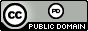시간 제한 메모리 제한 제출 정답 맞은 사람 정답 비율
1 초 1024 MB 3 1 1 33.333%

## 문제

The scientists working at the Family Genetics Institute are tracing the spread of a hereditary disease through family trees.  They have started by listing the family members that have the disease, and the parent who passed the disease on to each child (we will assume that each child gets the disease from only one parent).  However, the scientists are confused about the names of different family relationships.  Parents, grandparents, and siblings they have a handle on.  But a relationship like "third cousin, twice removed" has been hard for them to wrap their heads around.   After much discussion they came up with some definitions that cleared things up.

Suppose we have two people conveniently named $A$ and $B$ and their closest common ancestor is named $C$ (what are the odds!).  We say that $A$ is $m$ generations removed from $C$\/ if there are $m$ direct descendants from $C$ ending with $A$.  Thus if $A$ is the daughter of $C$ she is $1$ generation removed; if she is the granddaughter of $C$ she is $2$ generations removed, and so on.  Any person is $0$ generations removed from themselves.

Now let $A$ be $m$ generations removed from $C$ and $B$ be $n$ generations removed from $C$ where $m \leq n$.  We can determine the relationship between $A$ and $B$ using the following rules:

1. if $m = 0$ then $B$ is the child of $A$ if $n=1$ or the $\mbox{great}^{n-2}$ grandchild of $A$ if $n > 1$.
2. if $0 < m = n$ then $A$ and $B$ are siblings if $n=1$ or $(n-1)$-th cousins if $n > 1$.
3. if $0 < m < n$ then $A$ and $B$ are $(m-1)$-th cousins $(n-m)$ times removed.

Notice that if $m = 1$ and $n = 2$ we get the interestingly named "$0$th cousins, $1$ time removed" for the relationships we typically describe as "aunt/uncle" or "niece/nephew".

Figure 1 below shows some examples for two new people named (what else) $D$ and $E$.

# of generations removed from common ancestor Relationship
$D$ $E$
$0$ $1$ $E$ is the child of $D$
$4$ $0$ $D$ is the great great grandchild of $E$
$3$ $3$ $D$ and $E$ are $2$nd cousins
$9$ $8$ $D$ and $E$ are $7$th cousins, $1$ time removed
$1$ $4$ $D$ and $E$ are $0$th cousins, $3$ times removed

Figure 1: Some example relationships

The scientists have given you the description of a family tree and pairs of people in the tree and have asked you to determine the relationships between members of each pair.

## 입력

Input begins with a line containing two positive integers $t$ $p$ ($t \leq 100, p \leq 1\,000$) specifying the  number of tree descriptions (described below) and the number of query pairs.  Following these are $t$ lines, each with one tree description.  Each tree description will be of the form $s_0$ $d$ $s_1$ $s_2 \ldots s_d$ indicating that person $s_0$ has $d$ children named $s_1$ through $s_d$.  All names are unique and contain only alphabetic characters.  Tree descriptions may be given in any order (i.e., the root of the entire tree may not necessarily be in the very first tree description).  No name will appear more than once as $s_0$ in the tree descriptions.  All the tree descriptions will combine to form exactly one tree, and the tree will have at least $2$ nodes and at most $100$ nodes.

Following this are $p$ lines of the form $s_i$ $s_j$ where $s_i \neq s_j$ and both names are guaranteed to be in the tree.

## 출력

Output the relationship for each pair of people, one per line, using the formats shown in Figure 1.  Always output $s_i$'s name first for each pair except when $s_j$ is the direct descendant of $s_i$ (as in the first example in Figure 1).  For the $n$-th ordinal number output $n$th except for $n=1,2,3,21,22,23,31,32,33,\ldots$  in which case you should output 1st, 2nd, 3rd, 21st, 22nd, 23rd, 31st, 32nd, 33rd, etc.  Also be sure to use times for all times removed except one, where you should use the word time.

## 예제 입력 1

4 5
Horatio 1 Irene
Chris 2 Gina Horatio
Alice 3 Dan Emily Frank
Bob 2 Alice Chris
Irene Bob
Dan Frank
Chris Emily
Alice Chris
Dan Irene


## 예제 출력 1

Irene is the great grandchild of Bob
Dan and Frank are siblings
Chris and Emily are 0th cousins, 1 time removed
Alice and Chris are siblings
Dan and Irene are 1st cousins, 1 time removed


## 예제 입력 2

4 6
A 4 B C D E
H 3 I J K
C 2 F G
D 1 H
G C
H A
F G
F H
F K
B K


## 예제 출력 2

G is the child of C
H is the grandchild of A
F and G are siblings
F and H are 1st cousins
F and K are 1st cousins, 1 time removed
B and K are 0th cousins, 2 times removed


## 출처• 문제를 만든 사람: Bob Roos